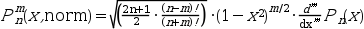Scilab Home page | Wiki | Bug tracker | Forge | Mailing list archives | ATOMS | File exchange
Scilab 5.5.0
Change language to: Français - Português - 日本語 - Русский

Scilab Help >> Special Functions > legendre

# legendre

associated Legendre functions

### Calling Sequence

`y = legendre(n,m,x [,normflag])`

### Arguments

n

non negative integer or vector of non negative integers regularly spaced with increment equal to 1

m

non negative integer or vector of non negative integers regularly spaced with increment equal to 1

x

real matrix(elements of `x` must be in the `[-1,1]` interval)

normflag

(optional) scalar string

### Description

When `n` and `m` are scalars, `legendre(n,m,x)` evaluates the associated Legendre function Pnm(x) at all the elements of `x`. The definition used is :where `Pn` is the Legendre polynomial of degree `n`. So `legendre(n,0,x)` evaluates the Legendre polynomial Pn(x) at all the elements of `x`.

When the normflag is equal to "norm" you get a normalized version (without the `(-1)^m` factor), precisely :which is useful to compute spherical harmonic functions (see Example 3):

For efficiency, one of the two first arguments may be a vector, for instance `legendre(n1:n2,0,x)` evaluates all the Legendre polynomials of degree n1, n1+1, ..., n2 at the elements of `x` and `legendre(n,m1:m2,x)` evaluates all the Legendre associated functions Pnm for m=m1, m1+1, ..., m2 at `x`.

### Output format

In any case, the format of `y` is :

`max(length(n),length(m)) x length(x)`

and :

```y(i,j) = P(n(i),m;x(j))   if n is a vector
y(i,j) = P(n,m(i);x(j))   if m is a vector
y(1,j) = P(n,m;x(j))      if both n and m are scalars```

so that `x` is preferably a row vector but any `mx x nx` matrix is expected and considered as an `1 x (mx * nx)` matrix, reshaped following the column order.

### Examples

```// example 1 : plot of the 6 first Legendre polynomials on (-1,1)
l = nearfloat("pred",1);
x = linspace(-l,l,200)';
y = legendre(0:5, 0,  x);
clf()
plot2d(x,y', leg="p0@p1@p2@p3@p4@p5@p6")
xtitle("the 6 th first Legendre polynomials")``````// example 2 : plot of the associated Legendre functions of degree 5
l = nearfloat("pred",1);
x = linspace(-l,l,200)';
y = legendre(5, 0:5, x, "norm");
clf()
plot2d(x,y', leg="p5,0@p5,1@p5,2@p5,3@p5,4@p5,5")
xtitle("the (normalized) associated Legendre functions of degree 5")``````// example 3 : define then plot a spherical harmonic
// 3-1 : define the function Ylm
function [y]=Y(l, m, theta, phi)
// theta may be a scalar or a row vector
// phi may be a scalar or a column vector
if m >= 0 then
y = (-1)^m/(sqrt(2*%pi))*exp(%i*m*phi)*legendre(l, m, cos(theta), "norm")
else
y = 1/(sqrt(2*%pi))*exp(%i*m*phi)*legendre(l, -m, cos(theta), "norm")
end
endfunction

// 3.2 : define another useful function
function [x, y, z]=sph2cart(theta, phi, r)
// theta row vector      1 x nt
// phi   column vector  np x 1
// r     scalar or np x nt matrix (r(i,j) the length at phi(i) theta(j))
x = r.*(cos(phi)*sin(theta));
y = r.*(sin(phi)*sin(theta));
z = r.*(ones(phi)*cos(theta));
endfunction

// 3-3 plot Y31(theta,phi)
l = 3; m = 1;
theta = linspace(0.1,%pi-0.1,60);
phi = linspace(0,2*%pi,120)';
f = Y(l,m,theta,phi);
[x1,y1,z1] = sph2cart(theta,phi,abs(f));       [xf1,yf1,zf1] = nf3d(x1,y1,z1);
[x2,y2,z2] = sph2cart(theta,phi,abs(real(f))); [xf2,yf2,zf2] = nf3d(x2,y2,z2);
[x3,y3,z3] = sph2cart(theta,phi,abs(imag(f))); [xf3,yf3,zf3] = nf3d(x3,y3,z3);

clf()
subplot(1,3,1)
plot3d(xf1,yf1,zf1,flag=[2 4 4]); xtitle("|Y31(theta,phi)|")
subplot(1,3,2)
plot3d(xf2,yf2,zf2,flag=[2 4 4]); xtitle("|Real(Y31(theta,phi))|")
subplot(1,3,3)
plot3d(xf3,yf3,zf3,flag=[2 4 4]); xtitle("|Imag(Y31(theta,phi))|")```